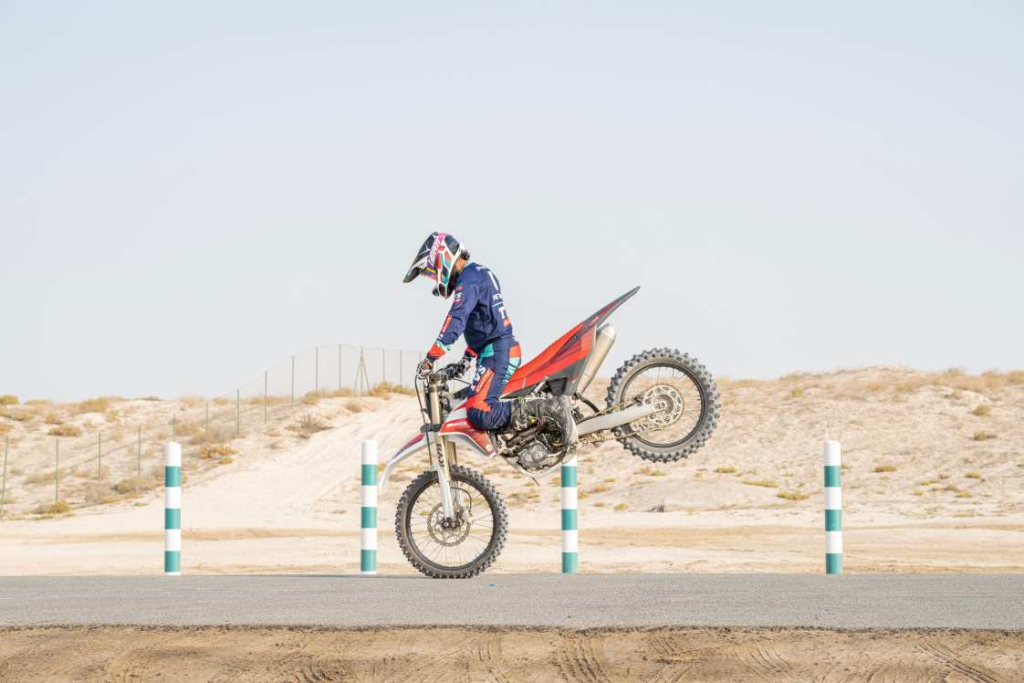# Probe Method – How to select features for ML models

The Probe method is a highly intuitive approach to feature selection. If a feature in the dataset contains only random numbers, it is not going to be a useful feature. Any feature that has lower feature importance than a random feature is suspicious.In this one, we will see:

## What is the Probe Method for Feature Selection?

The idea is to introduce a random feature to the dataset and train a machine learning model. This random feature is understand to have no useful information to predict the Y. After training the ML model, extract the feature importances.

The features that has lower feature importance scores compared to the random variable, are considered as weak and useless.

Drop the weak features.

Then reintroduce the random feature into the dataset and retrain the model to extract the feature importance scores. Again find out the variables that are weaker than the random variable. Repeat this process until you are left with zero variables to drop.

This is exactly how the probe method works. This is extremely intuitive, so it is easy to explain to your clients.

Which algorithm to use to train the model in Probe method?

Good question. It does not really matter. You can either go for the traditional logistic regression based model or use the algorithm that you are going to use to ultimately train your model.

1. Lesser variables implies shorter model training and inference
2. Easy to interpret.
3. Easier to train models on large datasets.
4. More reliable model perforance, since the poor variables are moved out.

## Install Feature Engine Package

The probe method is readily implemented in the feature-engine package. So, let’s use that for easy use.

First let’s install fearure-engine package.

# !pip install feature-engine==1.6.2
!python -c "import feature_engine; print('Feature Engine Version: ', feature_engine.__version__)"

Feature Engine Version:  1.6.2


## Import Packages

Mainly importing Logistic Regression and ProbeSelectionSelection.

# Import necessary libraries
import numpy as np
from sklearn import datasets
from sklearn.linear_model import LogisticRegression
from sklearn.model_selection import train_test_split

# Probe Method from FeatureEngine
from feature_engine.selection import ProbeFeatureSelection
import warnings
warnings.filterwarnings('ignore')


## Load Dataset and prepare train and test

Load dataset and train test split it.

# Load data
X = bc.data
y = bc.target
features = bc.feature_names

# Split data into training and test sets
X_train, X_test, y_train, y_test = train_test_split(X, y, test_size=0.2, random_state=42)


mean radius mean texture mean perimeter mean area mean smoothness mean compactness mean concavity mean concave points mean symmetry mean fractal dimension worst radius worst texture worst perimeter worst area worst smoothness worst compactness worst concavity worst concave points worst symmetry worst fractal dimension
68 9.029 17.33 58.79 250.5 0.10660 0.14130 0.31300 0.04375 0.2111 0.08046 10.31 22.65 65.50 324.7 0.14820 0.43650 1.25200 0.17500 0.4228 0.11750
181 21.090 26.57 142.70 1311.0 0.11410 0.28320 0.24870 0.14960 0.2395 0.07398 26.68 33.48 176.50 2089.0 0.14910 0.75840 0.67800 0.29030 0.4098 0.12840
63 9.173 13.86 59.20 260.9 0.07721 0.08751 0.05988 0.02180 0.2341 0.06963 10.01 19.23 65.59 310.1 0.09836 0.16780 0.13970 0.05087 0.3282 0.08490
248 10.650 25.22 68.01 347.0 0.09657 0.07234 0.02379 0.01615 0.1897 0.06329 12.25 35.19 77.98 455.7 0.14990 0.13980 0.11250 0.06136 0.3409 0.08147
60 10.170 14.88 64.55 311.9 0.11340 0.08061 0.01084 0.01290 0.2743 0.06960 11.02 17.45 69.86 368.6 0.12750 0.09866 0.02168 0.02579 0.3557 0.08020

5 rows × 30 columns

## Probe Feature Selection

Apply Probe Feature Selection method.

sel = ProbeFeatureSelection(
estimator=LogisticRegression(),
scoring="roc_auc",
n_probes=1,
distribution="uniform",
cv=3,
random_state=150,
)

X_tr = sel.fit_transform(X, y)


## Extract Feature Importances from the Probe Method

dict(round(sel.feature_importances_, 3))
{k: round(v, 3) for k, v in sorted(sel.feature_importances_.items(), key=lambda item: -item)}

{'worst radius': 1.022,
'worst concavity': 0.679,
'worst compactness': 0.552,
'texture error': 0.459,
'worst texture': 0.375,
'mean perimeter': 0.282,
'worst perimeter': 0.244,
'mean concavity': 0.243,
'mean texture': 0.236,
'worst concave points': 0.202,
'perimeter error': 0.19,
'mean compactness': 0.174,
'worst symmetry': 0.162,
'uniform_probe_0': 0.107,
'mean concave points': 0.105,
'area error': 0.101,
'worst smoothness': 0.069,
'worst fractal dimension': 0.055,
'mean symmetry': 0.05,
'concavity error': 0.048,
'mean smoothness': 0.038,
'compactness error': 0.035,
'mean area': 0.016,
'worst area': 0.016,
'concave points error': 0.013,
'symmetry error': 0.012,
'mean fractal dimension': 0.01,
'fractal dimension error': 0.003,
'smoothness error': 0.003}


## What Features to Drop?

We can safely drop the features that has lesser importance score comparatively.

sel.features_to_drop_

['mean area',
'mean smoothness',
'mean concave points',
'mean symmetry',
'mean fractal dimension',
'area error',
'smoothness error',
'compactness error',
'concavity error',
'concave points error',
'symmetry error',
'fractal dimension error',
'worst area',
'worst smoothness',
'worst fractal dimension']


## Probe Feature Selection using RandomForest

Let’s understand how Probe Selection performs on a RandomForest model. Is it giving the same set of feature?

from sklearn.ensemble import RandomForestClassifier

rfprobe = ProbeFeatureSelection(
estimator=RandomForestClassifier(),
scoring="roc_auc",
n_probes=1,
distribution="uniform",
cv=3,
random_state=150,
)

X_rf = rfprobe.fit_transform(X, y)

dict(round(rfprobe.feature_importances_, 3))
{k: round(v, 3) for k, v in sorted(rfprobe.feature_importances_.items(), key=lambda item: -item)}

{'worst perimeter': 0.135,
'worst concave points': 0.126,
'worst area': 0.097,
'mean concave points': 0.081,
'mean perimeter': 0.062,
'mean concavity': 0.059,
'area error': 0.05,
'worst concavity': 0.042,
'mean area': 0.041,
'worst texture': 0.017,
'worst compactness': 0.017,
'perimeter error': 0.015,
'mean texture': 0.015,
'worst smoothness': 0.012,
'worst symmetry': 0.011,
'mean compactness': 0.009,
'concavity error': 0.007,
'worst fractal dimension': 0.007,
'mean smoothness': 0.006,
'smoothness error': 0.005,
'compactness error': 0.005,
'fractal dimension error': 0.004,
'texture error': 0.004,
'symmetry error': 0.004,
'mean fractal dimension': 0.004,
'concave points error': 0.004,
'mean symmetry': 0.003,
'uniform_probe_0': 0.002}


Features to Drop according to Random Forest based Probe method

rfprobe.features_to_drop_


## Similar Articles

### Logistic Regression – A Complete Tutorial With Examples in RCourse Preview

## Machine Learning A-Z™: Hands-On Python & R In Data Science

### Free Sample Videos:#### Machine Learning A-Z™: Hands-On Python & R In Data Science#### Machine Learning A-Z™: Hands-On Python & R In Data Science#### Machine Learning A-Z™: Hands-On Python & R In Data Science#### Machine Learning A-Z™: Hands-On Python & R In Data Science